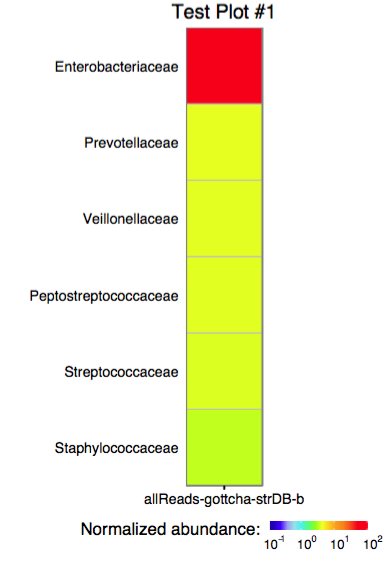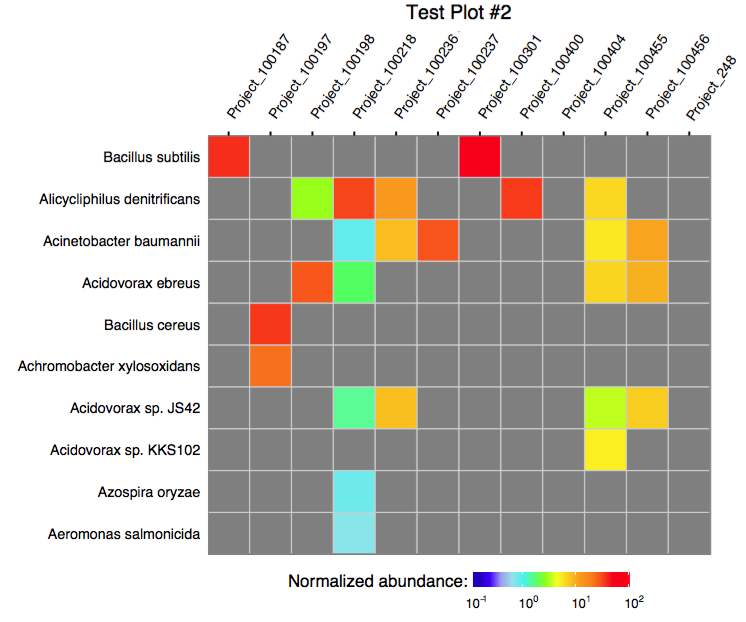# EDGE Taxonomy Assignments Visualization

Implements routines for metagenome sample taxonomy assignments collection, aggregation, and visualization. Accepts the EDGE-formatted output from GOTTCHA/GOTTCHA2, BWA, Kraken, MetaPhlAn, DIAMOND, and Pangia. Produces SVG and PDF heatmap-like plots comparing taxa abundances across projects.

Metagenome taxonomy assignment comparison toolkit. The toolkit is being developed for EDGE platform and reflects its backend specificity. The routines, however, can be used as a stand-alone library for multi-project comparative visualization of taxonomy assignments obtained for metagenomic samples processed with GOTTCHA/GOTTCHA2, BWA, KRAKEN, METAPHLAN, DIAMOND, or PANGIA. The heatmaps can be also visualized with this D3.js-based code which allows to see the exact abundance values in each cell.

#### 0.0 Installation from CRAN

``````install.packages("MetaComp")
``````

to use the library, simply load it into R environment:

``````library(MetaComp)
``````

#### 0.1 Installation from latest sources

``````install.packages("devtools")
library(devtools)
install_github(repo = 'seninp-bioinfo/MetaComp')
``````

#### 1.0 Reading a single taxonomic assignment files

``````the_gottcha2_assignment <- load_edge_assignment(data_file_g2, type = 'gottcha2')
the_kraken_assignment <- load_edge_assignment(data_file_k, type = 'kraken')
the_pangia_assignment <- load_edge_assignment(data_file_p, type = 'pangia')
``````

#### 1.1 Reading multiple taxonomic assignment files

The package functions `load_xxx_assignments` (where `xxx` stands for gottcha, kraken, or metaphlan) are designed to read a tool-specific assignment files. The configuration file for these functions must be tab-delimeted two columns file where the first column is the project id (used as the project's name in plotting), and the second column is an actual assignment file path:

``````the_assignments_list_g2 <- load_edge_assignments(config_file_g2, type = 'gottcha2')
the_assignments_list_k <- load_edge_assignments(config_file_k, type = 'kraken')
the_assignments_list_p <- load_edge_assignments(config_file_pangia, type = 'pangia')
``````

#### 2.0 Merging multiple taxonomic assignments into a single table

The `merge_edge_assignments` function is capable to merge a named list of GOTTCHA, Kraken, or MetaPhlAn assignments into a single table using `LEVEL` and `TAXA` columns as ids.

#### 3.0 Plotting a single assignment as a heatmap

The function `plot_edge_assignment` accepts a single assignment table and outputs a ggplot object or produces a PDF plot using ggplot2's `geom_tile`.#### 3.1 Plotting multiple assignments as a single heatmap

The function `plot_merged_assignment` accepts a single merged assignment table as an input and outputs a ggplot object or produces a PDF plot using ggplot2's `geom_tile`.#### 4.0. Running merge in a batch mode

The following script can be used to run the merge procedure in a batch mode:

``````# load library
require(MetaComp)
#
# configure runtime
options(echo = TRUE)
args <- commandArgs(trailingOnly = TRUE)
#
# print provided args
print(paste("provided args: ", args))
#
# acquire values
srcFile <- args
destFile <- args
taxonomyLevelArg <- args
plotTitleArg <- args
plotFileArg <- args
#
# extended functionality was added in the release #3, and we don't want to break the legacy systems
#
if (length(args) > 5) {
rowLimitArg <- args
sortingOrderArg <- args
} else {
rowLimitArg <- 60
sortingOrderArg <- "abundance"
}
#
# read the data and produce the merged table
merged <- merge_edge_assignments(load_edge_assignments(srcFile, type = "gottcha2"))
#
# write the merge table as a TAB-delimeted file
write.table(merged, file = destFile, col.names = T, row.names = F, quote = T, sep = "\t")
#
# produce a PDF of the merged assignment
plot_merged_assignment(assignment = merged, taxonomy_level = taxonomyLevelArg,
sorting_order = sortingOrderArg, row_limit = base::strtoi(rowLimitArg),
plot_title = plotTitleArg, filename = plotFileArg)
``````

To execute the scrip, use Rscript as shown below:

``````\$> Rscript merge_and_plot_gottcha_assignments.R assignments_table_gottcha.txt merged_assignments.txt \
family "Merge test plot" merge_test 20 alphabetical
``````

this command line arguments are (some of these are clickable -- so you can see examples):

# Reference manual

install.packages("MetaComp")

1.1.2 by Pavel Senin, 3 years ago

https://github.com/seninp-bioinfo/MetaComp

Report a bug at https://github.com/seninp-bioinfo/MetaComp/issues

Browse source code at https://github.com/cran/MetaComp

Authors: Pavel Senin [aut, cre]

Documentation:   PDF Manual